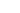## Learn C GamesThis blog is about C and Games programming (in C mainly) and now C# on mobile with MonoGame. It’s written by David Bolton, author of the Learn C Games Programming for beginners EBook. This is the Windows version, with a Raspberry Pi/Linux one due out isometime n 2021.

The first 20 chapters introduce and teach C programming with many examples. This link is to an .mp4 of the asteroids game from the book. It’s about 90 seconds long and demonstrates all of the features of the game. High score table, rotating asteroids (four sizes), sound, explosions, ship hyper-jump and shields.

The remaining 30 chapters builds up to full source code, about 2,000 lines, in 13 stages and I explain how each feature works and is implemented.  All of the book’s source code is on GithubMore about me. Buy it on Amazon(UK), Amazon(US).## Restarting the Raspberry PI C Games tutorials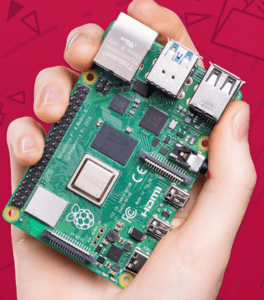I believe that the Raspberry PI, especially the 4B is a great and very low cost machine for not only running games but for developing them as well. Of course, if you have a PC, Linux or Mac then you can use that as a development machine but if you haven’t, it costs less than £100 (when you count the system, case, cables, SD-Card) to get up and running.

So I’m reworking my original eBook for the Raspberry Pi, using software running on the Pi and developing a 2nd ebook. Along the way I’ll publish longer excerpts from it here. Probably one a week.## Interesting looking Game Handheld device- Odroid-GoThe Odroid-go Game kit looks like a gameboy. It has a LCD, game controllers and the case. It has a CPU that runs between 80 and 240 MHZ, 4MB RAM, WiFi, Bluetooh, a Micro-SD slot, a rechargeable battery – charged through a Micro USB, a built-in speaker and a 320 x 240 LCD. That may not sound very much but all the CBM-64 games ran on that size of screen and it only had 64 KB of RAM.

There’s more information on the manufacturers website (it’s in English though it is Chinese made) and all the useful information is on a Wiki with info on emulators, downloadable games and how to write programs for it using the Arduino programming language which is very much like C/C++. You have to install Arduino for ESP32 first on a Pc (Windows, Linux or Mac) then can you write programs and run them on your Odroid-Go.

There’s a bit of assembling but the website includes instructions with photos. A quick search on Youtube found a video showing how to assemble it.

This is an example of Arduino code, taken from their online reference manual. The Ardunio has been around for several years so there is a lot of code around for it.

``````// the setup function runs once when you press reset or power the board
void setup() {
// initialize digital pin LED_BUILTIN as an output.
pinMode(LED_BUILTIN, OUTPUT);
}

// the loop function runs over and over again forever
void loop() {
digitalWrite(LED_BUILTIN, HIGH);   // turn the LED on (HIGH is the voltage level)
delay(1000);                       // wait for a second
digitalWrite(LED_BUILTIN, LOW);    // turn the LED off by making the voltage LOW
delay(1000);                       // wait for a second
}``````## More on Shuffledness

So I’ve updated my shuffledness calculation. This is a bit of a work in progress so probably isn’t the last version.  One of the things I’m interested in is what is the optimal number of swaps to get a good shuffled deck of cards. The shuffle algorithm picks two random indexes in the array 0-51 and then swaps the contents.

Obviously doing this 10 times would only shuffle at most 20 cards and probably fewer as there’s no checks for repeats. The only check is that both indexes (indices?) are not the same. So I want to experiment and see what an optimal value for numTries should be..

``````void ShuffleDeck(int numtries) {
int firstIndex, secondIndex;
for (int i = 0; i < numtries; i++) {
do {
firstIndex = rand() % 52;
secondIndex = rand() % 52;
}
while (firstIndex == secondIndex);
int value = deck[firstIndex];
deck[firstIndex] = deck[secondIndex];
deck[secondIndex] = value;
}
}``````

Anyway, I’ve added a CalcDistance() function. This looks at each card and calculates how far it has moved from it’s original position.  It then divides this by the maximum distance it could have moved to get a move “factor”. These are summed up and divided by 52 to give an average move factor. That’s your CalcDistance() function.

``````float CalcDistance() {
float distance = 0.0f;
for (int i = 0; i < 52; i++) {
float distanceDiv = (i < 26) ? 51 - i : i-1;
//printf("%i: %3.0f\n", i, distanceDiv);
distance += (abs(deck[i] - i) / distanceDiv);
}
return distance/52.0f;
}``````

The only thing odd about this function is the distanceDiv value which starts at 51, decreases to 25 then increases to 50. The printf let me check that was correct.

This is the current state of the program which I’ve listed fully below. Enjoy! It’s 63 lines long.

``````// shuffledness.c : This measures how shuffled a deck of cards is
// cards are hjeld as values 0-51 in a 52 int aaray.

#include <time.h>
#include <stdio.h>
#include <stdlib.h>

int deck;
time_t t;

// Order the cards 0-51. 0 = Acew Hards,13 = Ace Clubs,26 = Ace of Diamonds and 51 = King of Spades
void InitDeck() {
for (int i = 0; i < 52; i++) {
deck[i] = i;
}
}

// Works on glovbal array deck and calculates a value for how displayed each card is
float CalcDisorder() {
int total=0;
for (int i = 1; i < 52; i++) {
total += abs(deck[i] - deck[i - 1]);
}
printf("Total = %d\n", total);
}

// Sum up distance card moved/max distance
float CalcDistance() {
float distance = 0.0f;
for (int i = 0; i < 52; i++) {
float distanceDiv = (i < 26) ? 51 - i : i-1;
//printf("%i: %3.0f\n", i, distanceDiv);
distance += (abs(deck[i] - i) / distanceDiv);
}
return distance/52.0f;
}

// Shuffle a deck by swapping two random cards a specified number of times
void ShuffleDeck(int numtries) {
int firstIndex, secondIndex;
for (int i = 0; i < numtries; i++) {
do {
firstIndex = rand() % 52;
secondIndex = rand() % 52;
}
while (firstIndex == secondIndex);
int value = deck[firstIndex];
deck[firstIndex] = deck[secondIndex];
deck[secondIndex] = value;
}
}

int main() {
/* Intializes random number generator */
srand((unsigned)time(&t));
for (int i = 0; i < 25; i++) {
InitDeck();
ShuffleDeck(1000);
printf("CalcDisorder() ==%f x %f = %f\n", CalcDisorder(),CalcDistance(),(double)CalcDisorder() * CalcDistance());
}
return 0;
}
``````

Because I’m multiplying the two factors (disorder and Distance, the final value is never getting much above 0.35 and I’d prefer it to be in the range 0-0.999. Suggestions welcomed.

.So, if you are new to programming Compiling and linking can seem like magic. The compiler transforms C source code files into obj files. The actual format is different depending on the CPU and Operating system.  Linking is even more magic because it takes a bunch of obj files and produces a single executable.

There’s actual more magic going on than my simplistic explanation. The compiler might be doing optimisation (generally in release mode but not debug). That makes debug compiles faster which is more desirable.

Another blog called Hack the developer has published an article with a lot more detail about compiling and linking. If you want to get a better understanding then this is a petty good piece. It uses Linux, GCC and Clang as examples. It also goes into more depth on the layout of obj files.## How to visit many many places in one go

No this isn’t turning into a travellog.  Imagine a huge square. It has 33,554,432 points along an edge. The entire square would thus have 1,125,899,906,842,642 points in it. That’s 250 or 3210 points. (Yes those two numbers are the same!)

Now how about contriving an algorithm that will pick a random point in this square and keep picking each point but never more than once? Eventually it will have picked every point.  If it could visit a million points every seconds, it would take something like 35.7 years to visit each and every point.

So assuming you could create an algorithm, how large would it be? How about three lines?

``````        public static ulong Nextulong()
{
ulong result = ((aUVal * ULast) + bUVal) % aUVal;
ULast = result;
return result;
}``````

That’s in C#. The values for aUVal and bUval are

``````        const ulong aUVal = 1125899906842597;
const ulong bUVal = 1051122009542795;``````

A uLong is just an unsigned long. That’s a 64 bit unsinged int.
This is an example of a Linear Congruential Generator BTW.## How to count lines of code in Visual Studio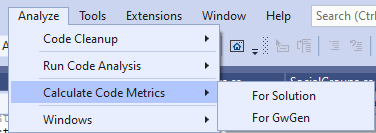Until this morning, I didn’t realise that Visual Studio (even the free Community Edition) has this built in. I was curious to see how much code I’d written so far for my game processing engine.  Development comes in leaps and bounds during evenings and weekends.

Visual Studio has Code Metrics in it. You can do this for any project that builds correctly. To show this you have to do View => Other Windows => Code Metrics Results. That displays the window. Then you have to go on the Analyze Window, and click Calculate Code Metrics.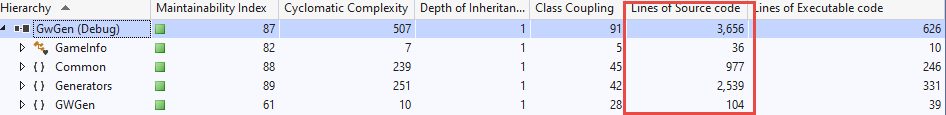However you might want to take these with a pinch of salt. As I understand it, Lines of Source Code is calculated from the il code output from the compiler. The last column (Lines of executable code) suggests that most of my program is comments which is wrong! There is however a fair amount of data in the form of Constructor initializations, and one file of 1290 lines has over 1,000 such initializations.  You can read what the other columns mean and everything you ever wanted to know about code metrics on the Microsoft site.

Out of curiosity, I manually counted the number of lines in this project. There are 15 files and they added up to 3700 lines but that includes comments and blank lines.  A quick search for // found 119 which probably means I should improve my commenting. Blank lines is probably something similar. So we’re talking 3700-1000 (constructor initializers)-119 (comments) -119 (blank lines) = 2462 lines of code so far. That’s working tested code mind you.## 39 Puzzles in C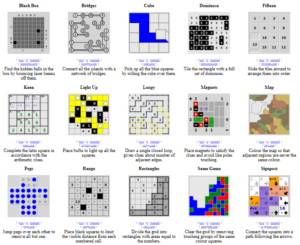Those of you who have used the Putty utility to connect to a remote computer using SSH might recognise the name chiark. It’s also the home of various puzzles that are coded in C and they’ve made the source code available.  You can read about each puzzle via the online documentation.

The puzzles are one player games and run natively on Unix (GTK), on Windows, and on Mac OS X. As usual I’ve added these to the C code links as well. Puzzles are near enough to games to count! I’ve screen grabbed 15 of them but there are almost 40 in total.

If you like this sort of thing and have an idea for a game, take a look at the devel page. The puzzles are split into three parts, front, middle and back ends. If you want to implement a new front end (SDL2 anyone?) then you just change the front end.## A better random number generator

How’s this for a minimal RNG implementation in C?

``````// *Really* minimal PCG32 code / (c) 2014 M.E. O'Neill / pcg-random.org

typedef struct { uint64_t state;  uint64_t inc; } pcg32_random_t;

uint32_t pcg32_random_r(pcg32_random_t* rng)
{
uint64_t oldstate = rng->state;
rng->state = oldstate * 6364136223846793005ULL + (rng->inc|1);
// Calculate output function (XSH RR), uses old state for max ILP
uint32_t xorshifted = ((oldstate >> 18u) ^ oldstate) >> 27u;
uint32_t rot = oldstate >> 59u;
return (xorshifted >> rot) | (xorshifted << ((-rot) & 31));
}``````

https://www.pcg-random.org/

is a website dedicated to random numbers using their algorithms, The whole site is good but I particularly like the party tricks page where they show that one of their random number generators generates a sequence that contains interesting things like source of a C file, zip files and text from hamlet.## More thoughts on shuffledness

Thinking about what I said, it can’t be just about how far a card moves when shuffled but how much disordering occurs as well. If you shuffled the deck and discovered 7 cards in a sequence like 2,3,4,5,6 of hearts deep into the deck, they would have moved from near the top of the deck (an “ordered deck” is A-K in Hearts, Clubs, Diamonds and Spades) then it probably wasn’t a very good shuffle.

One way to measure “disorder” is to subtract each card’s ordinal value from it’s successor and sum up the absolute value of them . Ordinal value is the value 0-51 where 0 = Ace Hearts, 1 = 2 Hearts and so on. In an ordered deck the sum of all these subtractions will be 51. In a well shuffled deck I would guess it might be something like 1352 (52 x 26). Absolute value is the abs(x) function of x which is always positive if x is negative.  abs(-8) = 8. abs(8) = 8.

So now for shuffledness we have two values. Displacement and disorder. If both are calculated as a value between 0 and 1 then multiplying them together might work as a measure of shuffledness.

Here’s a very simple example of calculating the value for disorder. So far the calculations have never got a total much above 900, and not near 1352. I’ll run a few experiments and see the range of values.

``````// shuffledness.c : This measures how shuffled a deck of cards is
// cards are held as values 0-51 in a 52 int array.

#include <time.h>
#include <stdio.h>
#include <stdlib.h>

int deck;
time_t t;

// Order the cards 0-51. 0 = Acew Hards,13 = Ace Clubs,26 = Ace of Diamonds and 51 = King of Spades
void InitDeck() {
for (int i = 0; i < 52; i++) {
deck[i] = i;
}
}

// Works on glovbal array deck and calculates a value for how displayed each card is
float CalcDisorder() {
int total=0;
for (int i = 1; i < 52; i++) {
total += abs(deck[i] - deck[i - 1]);
}
printf("Total = %d\n", total);
}

// Shuffle a deck by swapping two random cards a specified number of times
void ShuffleDeck(int numtries) {
int firstIndex, secondIndex;
for (int i = 0; i < numtries; i++) {
do {
firstIndex = rand() % 52;
secondIndex = rand() % 52;
}
while (firstIndex == secondIndex);
int value = deck[firstIndex];
deck[firstIndex] = deck[secondIndex];
deck[secondIndex] = value;
}
}

int main() {
/* Intializes random number generator */
srand((unsigned)time(&t));
InitDeck();
ShuffleDeck(1000);
printf("CalcDisorder() == %f\n", CalcDisorder());
return 0;
}``````

That’s about 50 lines long. I’ll work on it and add the displacement calculation and try and get a maximum figure the the disorder calculation.## Visual Studio Code Now supports ARM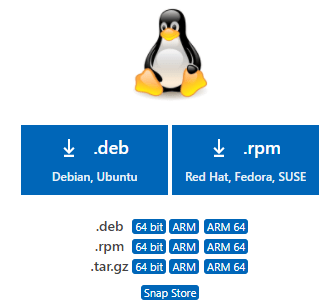Which means it supports Raspberry Pi. Of course I’ve been using it thanks to the code.headmelted.com version but it’s convenient now that both 32-bit and 64-bit ARM are available to download from the official site. As always, make sure you pick the .deb version either ARM for the 32-bit Raspberry Pi OS or ARM 64 if you have the 64-bit.

Apparently there is also a new C/C++ extension from Microsoft that I shall have to check out as well.

Also this means you can do remote code development. The idea is that you run Visual Studio Code on your PC/Mac whatever and connect to a program running on your PI (over WifI or a Network cable) using SSH. See the VS Code documentation for remote development.